#Quants : Quiz From Mock Test 5 with Explanation

Hello friends,This is quiz from SBI PO mock test (Quants section ) with explanation.Numbering of questions is as per numbering in mock test 5

16)The cost of 10 kg of apples equals the cost of 24 kg of rice. The cost of 6 kg of flour equals the cost of 2 kg of rice. The cost of each kg of flour is Rs.20.50. Find the total cost of 4 kg of apples, 3 kg of rice and 5 kg of flour.
1 : Rs.849.40
2 : Rs.877.40
3 : Rs.901.60
4 : Rs.815.20
5 : None of these

2 : Rs.877.40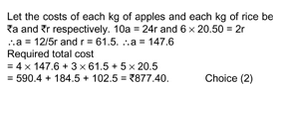17)Ravi purchased a refrigerator and a mobile phone at Rs.15000 and Rs.8000 respectively. He sold the refrigerator at a loss of 4 percent and the mobile phone at a profit of 10 percent. On the whole, he got a ____.
1 : loss of Rs.200
2 : loss of Rs.100
3 : profit of Rs.100
4 : profit of Rs.200
5 : None of these

4 : profit of Rs.200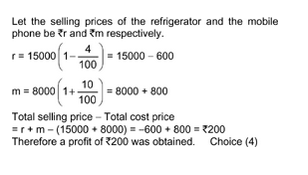18)By how much percent is 3/16 of 368 less than 12.5% of 736?
1 : 33 1/3%
2 : 66 2/3%
3 : 20%
4 : 75%
5 : 25 %

5 : 25 %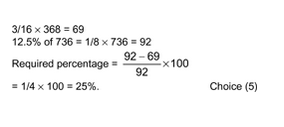19)The perimeter of a square is equal to the perimeter of a rectangle. The area of the rectangle is 56 sq cm. What is the area of the square?
1 : 64 sq cm
2 : 49 sq cm
3 : 36 sq cm
4 : Cannot be determined
5 : None of these

4 : Cannot be determined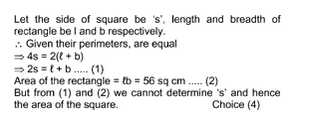20)A can do a job in 4 days and B can do the same job in 6 days. If A’s efficiency increases to 4/3 times, then by how many times B has to reduce his efficiency so that the work can be completed by both of them in two days?
1 : 2/3 times
2 : 2 times
3 : 4/3times
4 : 1/2times
5 : None of these

5 : None of these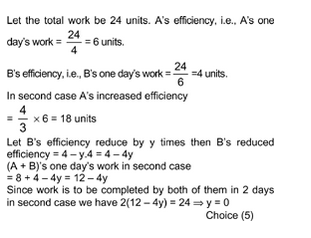21)A canteen requires 210 kg of wheat for 2 weeks. How many kg of wheat will it require for 116 days at the same rate?
1 : 812
2 : 1740
3 : 1416
4 : 1540
5 : None of these

2 : 1740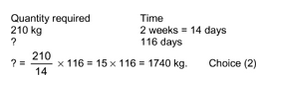22)A candidate who appeared for an examination had to secure 35% marks to pass. He secured 80 marks and failed by 60 marks. What must be the maximum mark in the examination?
1 : 560
2 : 360
3 : 400
4 : 300
5 : 420

3 : 400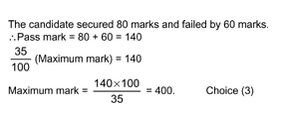23)A sum of Rs.4500 amounts to Rs.5544 in two years at compound interest compounded annually. If the rate of interest for the first year is 12% p.a., find the rate of interest for the second year.
1 : 10% p.a.
2 : 12% p.a.
3 : 15% p.a.
4 : 20% p.a.
5 : None of these

1 : 10% p.a.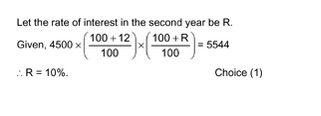24)The average marks obtained by 25 students in a class was calculated as 52. But the marks of one student was misread as 61 instead of 16. What is the actual average?
1 : 53.8
2 : 50.2
3 : 72
4 : 50.8
5 : None of these

2 : 50.2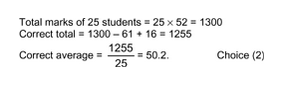25) Three friends Ramesh, Suresh and Harish started a business by investing Rs.2,65,000 in the ratio of 12 : 15 : 26. Ramesh stayed in the business for six months, Suresh for eight months and Harish stayed in the business till the end of first year. If they earned a profit of Rs.42,000 then what is the share of Harish in the profit?
1 : Rs.20,000
2 : Rs.22,000
3 : Rs.24,000
4 : Rs.26,000
5 : None of these

4 : Rs.26,000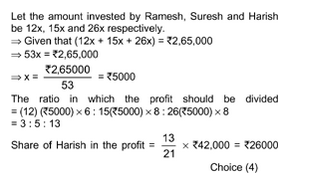26)A bag contains 5 red balls, 3 black balls and a white ball. If three balls are drawn at random, the probability that the three balls are of different colours is ____.
1 : 23/28
2 : 5/28
3 : 3/28
4 : 3/14
5 : None of these

2 : 5/28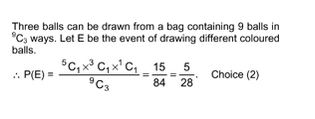27)An urn contains 4 green, 3 red and 2 brown marbles. If two marbles are picked up at random, what is the probability that both of them are either green or brown?
1 : 11/36
2 : 1/6
3 : 7/36
4 : 5/12
5 : None of these

4 : 5/12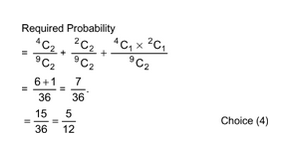28) A and B together can complete a piece of work in 8 days. B and C together can complete the same work in 12 days. C and A together can complete the same work in 16 days. A and B began the work together and left after some days. C finished the remaining work. If it took a total of 74 days to complete the entire work, for how many days did A and B work together?
1 : 3
2 : 4
3 : 2
4 : 6
5 : None of these

3 : 2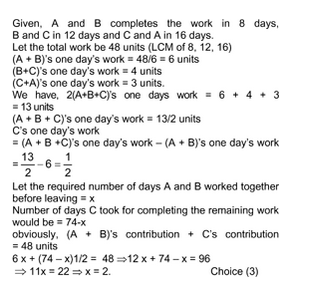29)A fraction which is in its lowest terms reduces to 1/2 if one is added to both the numerator and the denominator and to 4/9 if 4 is subtracted from both the numerator and the denominator. Then which of the following does the fraction reduce to when 6 is added to both the numerator and the denominator?
1 : 7/12
2 : 3/8
3 : 6/11
4 : 2/13
5 : None of these

3 : 6/11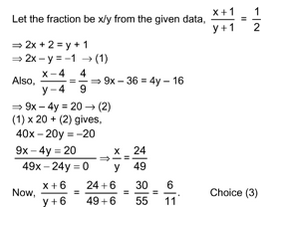30)Keshav sold an article at five-fourth of its cost price. What discount percent he must offer on the selling price, if he wanted to earn a profit of 15%?
1 : 16%
2 : 10%
3 : 12%
4 : 8%
5 : None of these

4 : 8%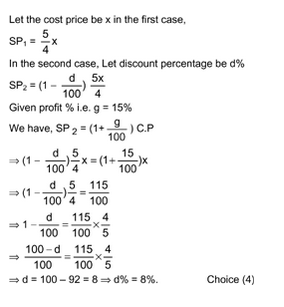AffairsCloud Recommends Oliveboard Mock Test

AffairsCloud Ebook - Support Us to Grow